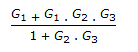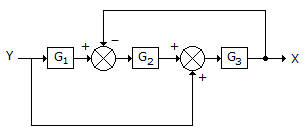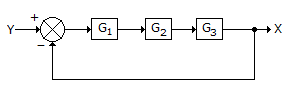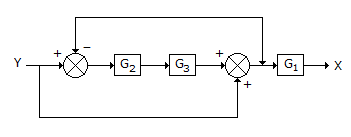# Chemical Engineering - Process Control and Instrumentation

### Exercise :: Process Control and Instrumentation - Section 3

6.

Pirani gauge is used for the measurement of

 A. very high pressure. B. high vacuum. C. liquid level under pressure. D. liquid level at atmospheric pressure

Explanation:

No answer description available for this question. Let us discuss.

7.

The __________ of the fluid contained in the temperature sensing element (i.e., bulb) of filled system thermometers changes with change in temperature.

 A. pressure B. volume C. viscosity D. all (a), (b) and (c)

Explanation:

No answer description available for this question. Let us discuss.

8.

Transfer functions X/Y for which of the following block diagrams isA.B.C.D. none of these

Explanation:

No answer description available for this question. Let us discuss.

9.

The characteristic equation for the control system with a closed loop transfer function G1/1 + G2 is given by 1 + G2 = 0. The characteristic equation for the control system

 A. depends only upon the open loop transfer function. B. determines its stability. C. is the same for set point or load changes. D. all 'a', 'b' & 'c'

Explanation:

No answer description available for this question. Let us discuss.

10.

__________ thermometer can not measure sub-zero (< 0° C) temperature,

 A. Mercury in glass B. Bimetallic C. Vapor pressure D. Resistance